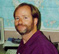# Spectral Flipping Around Signal Center Frequency

Most of us are familiar with the process of flipping the spectrum (spectral inversion) of a real signal by multiplying that signal's time samples by (-1)n. In that process the center of spectral rotation is fs/4, where fs is the signal's sample rate in Hz. In this blog we discuss a different kind of spectral flipping process.

Consider the situation where we need to flip the X(f) spectrum in Figure 1(a) to obtain the desired Y(f) spectrum shown in Figure 1(b). Notice that the center of rotation of the desired spectral flipping is not fs /4, but is instead the signal's fcntr center frequency. The simple spectral flipping process of multiplying a signal's time samples by (-1)n does not solve our problem because that process would result in the undesirable spectrum shown in Figure 1(c).Figure 1: Spectral flipping, centered at fcntr: (a) original spectrum ranging from fLow to fHigh; (b) desired spectrum; (c) incorrect spectrum.

There are two methods to solve our fcntr-centered spectral flipping problem. Figure 2(a) shows the first method, comprising a multirate processing technique. In considering this spectral flipping method, we should keep in mind that:

* The two identical lowpass filters have passbands that extend from zero Hz to fHigh Hz. (Note that the sample rate for both filters is 2fs Hz.)

* The cosine mixing sequence uses the upsampled-by-two time index variable n'.

* The multiply by four operation compensates for the sequence u(n') amplitude loss by a factor of two caused by interpolation, and the amplitude loss by another factor of two due to the cosine mixing.

Of course, a clever engineer will eliminate the multiply by four operation altogether by increasing the DC (zero Hz) gain of one of the lowpass filters by four.

The second method we could use to obtain a signal having the desired Figure 1(b) spectrum is the neat process shown in Figure 2(b), suggested by Dirk Bell . While somewhat more computationally intensive than the above multirate method, this technique works well and deserves mention here. In this second method, as Bell recommends, we can combine the last two stages of the process (the complex multiply and the Real Part extraction) by computing only the real part of sequence v(n) yielding our desired y(n).Figure 2: Spectral flipping techniques: (a) first method; (b) second method.References:

 Bell, D. "Subject: Re: Frequency Inversion", Usenet group comp.dsp post, Aug. 30, 2006.

[ - ]
Comment by November 24, 2007Hi Rick, Interesting technique. I saw this done with analog circuits about 25 years ago for police radio communications. Someone had the idea that if you flipped the spectrum of the voice (exchanging the high and low frequencies) that eavesdroppers would not be able to understand it. Didnât work very wellâ people could actually learn to understand the inverted sound! Thanks for the article.
[ - ]
Comment by December 26, 2007thnx rick this is great
[ - ]
Comment by March 31, 2009Hi Rick, For the first way, there is a little backword when the spectrum of baseband you want to flip is very close to zero, for example, BW = [0 0.25Fs], f_Low = 0; f_High = 0.25Fs, then it will become very hard to do the second LPF, isn't it?
[ - ]
Comment by April 1, 2009Hi xie.qiang, Yes, you are correct. The second LPF's transition-region width must be kept smaller than 2*fLOW (2 times fLOW). So if fLOW is small, the second LPF will have many taps (high order). Good Luck, [-Rick Lyons-]

To post reply to a comment, click on the 'reply' button attached to each comment. To post a new comment (not a reply to a comment) check out the 'Write a Comment' tab at the top of the comments.